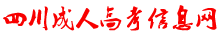# 2020年广东成人高考文科数学考点：求解函数解析式

求解函数解析式是高考重点考查内容之一，需引起重视.本节主要帮助考生在深刻理解函数定义的基础上，掌握求函数解析式的几种方法，并形成能力，并培养考生的创新能力和解决实际问题的能力。

●难点磁场

(★★★★)已知f(2-cosx)=cos2x+cosx,求f(x-1).

●案例探究

[例1](1)已知函数f(x)满足f(logax)= (其中a>0,a≠1,x>0),求f(x)的表达式.

(2)已知二次函数f(x)=ax2+bx+c满足|f(1)|=|f(-1)|=|f(0)|=1,求f(x)的表达式.

命题意图：本题主要考查函数概念中的三要素：定义域、值域和对应法则，以及计算能力和综合运用知识的能力.属★★★★题目.

知识依托：利用函数基础知识，特别是对“f”的理解，用好等价转化，注意定义域.

错解分析：本题对思维能力要求较高，对定义域的考查、等价转化易出错.

技巧与方法：(1)用换元法;(2)用待定系数法.

解：(1)令t=logax(a>1,t>0;0

因此f(t)= (at-a-t)

∴f(x)= (ax-a-x)(a>1,x>0;0

(2)由f(1)=a+b+c,f(-1)=a-b+c,f(0)=c

得 并且f(1)、f(-1)、f(0)不能同时等于1或-1，所以所求函数为：f(x)=2x2-1或f(x)=-2x2+1或f(x)=-x2-x+1或f(x)=x2-x-1或f(x)=-x2+x+1或f(x)=x2+x-1.

[例2]设f(x)为定义在R上的偶函数，当x≤-1时，y=f(x)的图象是经过点(-2，0)，斜率为1的射线，又在y=f(x)的图象中有一部分是顶点在(0，2)，且过点(-1，1)的一段抛物线，试写出函数f(x)的表达式，并在图中作出其图象.

命题意图：本题主要考查函数基本知识、抛物线、射线的基本概念及其图象的作法，对分段函数的分析需要较强的思维能力.因此，分段函数是今后高考的热点题型.属★★★★题目. 知识依托：函数的奇偶性是桥梁，分类讨论是关键，待定系数求出曲线方程是主线.

错解分析：本题对思维能力要求很高，分类讨论、综合运用知识易发生混乱.

技巧与方法：合理进行分类，并运用待定系数法求函数表达式.

解：(1)当x≤-1时，设f(x)=x+b

∵射线过点(-2，0).∴0=-2+b即b=2，∴f(x)=x+2.

(2)当-1

∵抛物线过点(-1，1)，∴1=a·(-1)2+2,即a=-1

∴f(x)=-x2+2.

(3)当x≥1时，f(x)=-x+2

综上可知：f(x)= 作图由读者来完成.

●锦囊妙计

本难点所涉及的问题及解决方法主要有：

1.待定系数法，如果已知函数解析式的构造时，用待定系数法;

2.换元法或配凑法，已知复合函数f[g(x)]的表达式可用换元法，当表达式较简单时也可用配凑法;

3.消参法，若已知抽象的函数表达式，则用解方程组消参的方法求解f(x);

另外，在解题过程中经常用到分类讨论、等价转化等数学思想方法.

#### 声明：

（一）由于考试政策等各方面情况的不断调整与变化，本网站所提供的考试信息仅供参考，请以权威部门公布的正式信息为准。

（二）网站文章免费转载出于非商业性学习目的，版权归原作者所有。如您对内容、版权等问题存在异议请与本站联系，我们会及时进行处理解决。

### 广东成考交流群### 扫一扫加入考生微信群

#### 最新信息榜